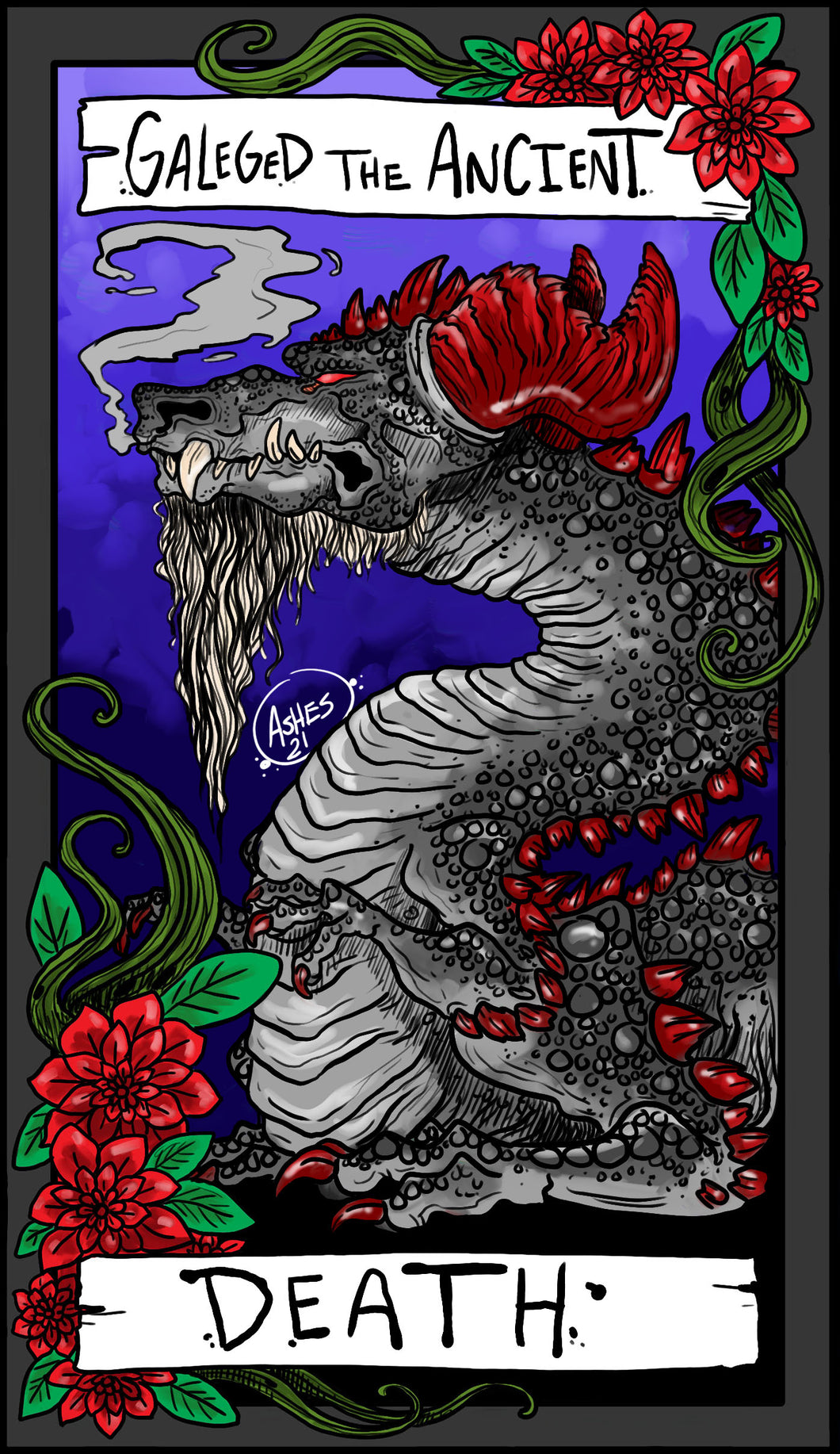# Galeged the Ancient

Galeged the Ancient Size Mini:

Circumference of tip: 3 inches

Circumference below head: 3 inches

Circumference of widest point of knot: 4 inches

Circumference below knot: 3.2 inches

4 inch usable length

5 inch total length

__________

Galeged the Ancient size Small:

Circumference of tip: 3.5 inches

Circumference below head: 4 inches

Circumference of widest point of knot: 5 inches

Circumference below knot: 4.3 inches

5.5 inch usable length

6.5 inch total length

__________

Galeged the Ancient size medium:

Circumference of tip: 4 inches

Circumference below head: 5 inches

Circumference of widest point of knot: 6.5 inches

Circumference below knot: 5.3 inches

7 inch usable length

8 inch total length

__________

Galeged the Ancient size Large:

Circumference of tip: 4.5 inches

Circumference below head: 6 inches

Circumference of widest point of knot: 7.5 inches

Circumference below knot: 6.5 inches

8 inch usable length

9.5 inch total length